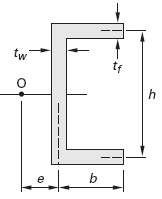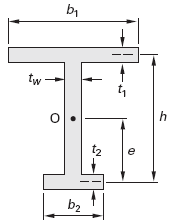Related Resources: calculators

### Shear Center for Beams Equations and Calculator

Shear Center for Beams Equations and Calculator

A beam with a symmetrical cross section supporting a transverse force that is offset from the longitudinal centroidal axis will be acted upon by a torsional moment, and the beam will tend to “roll” about a longitudinal axis known as the bending axis or torsional axis. For solid, symmetrical cross sections, this bending axis passes through the centroid of the cross section. However, for an asymmetrical beam (e.g., a channel beam on its side), the bending axis passes through the shear center (flexural center, torsional center, or center of twist), not the centroid. (See Fig. 1.) The shear center is a point that does not experience rotation (i.e., is a point about which all other points rotate) when the beam is in torsion.Figure 1, Channel Beam in Pure Bending
(shear resultant, V, directed through shear center, O)

For beams with transverse loading, simple bending without torsion can only occur if the transverse load (shear resultant, V, or shear force of action) is directed through the shear center. Otherwise, a torsional moment calculated as the product of the shear resultant and the torsional eccentricity will cause the beam to twist. The torsional eccentricity is the distance between the line of action of the shear resultant and the shear center.

The shear center is always located on an axis of symmetry. The location of the shear center for any particular beam geometry is determined by setting the torsional moment equal to the shear resisting moment, as calculated from the total shear flow and the appropriate moment arm. In practice, however, shear centers for common shapes are located in tables similar to Fig. 2.

Shear Centers of Selected Thin-Walled Open Sections*

Simple Channel Shear Center EquationEq. 1
e = ( 3 · tf · b2 ) / ( h · tw + 6 · b · tf )

"C" Section Shear Center Equation

Open "C" Section Shear Center CalculatorEq. 2
e = b [ ( 3 · h2 · b + 6 · h2 · c - 8 · c3 ) / ( h3 + 6 · h2 · b + 6 · h2 · c + 8 · c3 - 12 · h · c2 ) ]

Modified "I" Section Shear Center Equation

Modified "I" Section Shear Center CalculatorEq. 3
e = ( t1 · b31 · h ) / ( t1 · b31 + t2 · b32 )

*Distance e from shear center, O, measured from the reference point shown. Distance e is not the torsional eccentricity, which is the separation of the shear center and the line of action of the shear force.

Reference

Civil Engineering Reference Manual, Fifteenth Edition, Michael R. Lindeburg, PE

Related